##### Geometry For Dummies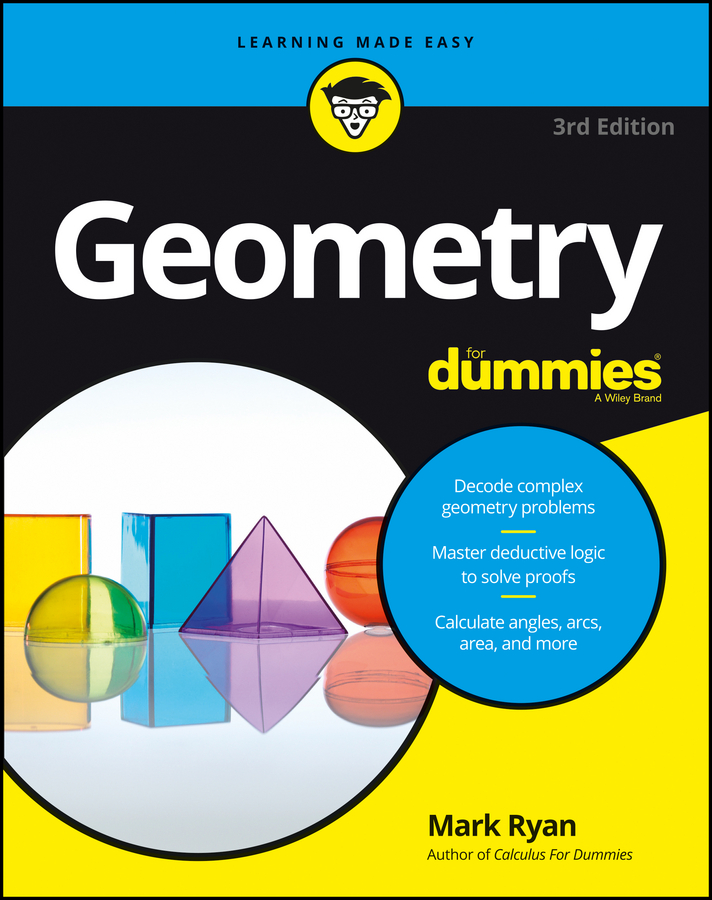You can use the AA (Angle-Angle) method to prove that triangles are similar. The AA theorem states that if two angles of one triangle are congruent to two angles of another triangle, then the triangles are similar. This is the most frequently used method for proving triangle similarity and is therefore the most important. Luckily, it’s also easy to use. Give it a whirl with the following proof:

to –2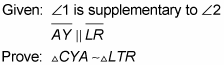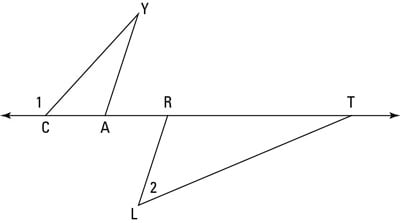Here’s a game plan describing how your thought process might go (this hypothetical thought process assumes that you don’t know that this is an AA proof): The first given is about angles, and the second given is about parallel lines, which will probably tell you something about congruent angles. Therefore, this proof is almost certainly an AA proof (as opposed to the other two methods of proving triangles similar both of which involve sides of the triangles). So all you have to do is think about the givens and figure out which two pairs of angles you can prove congruent to use for AA. Duck soup.

Take a look at how the proof plays out:

Statement 1: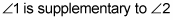Reason for statement 1: Given.

Statement 2: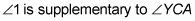Reason for statement 2: Two angles that form a straight angle (assumed from diagram) are supplementary.

Statement 3: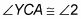Reason for statement 3: Supplements of the same angle are congruent.

Statement 4: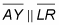Reason for statement 4: Given.

Statement 5: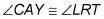Reason for statement 5: Alternate exterior angles are congruent (using parallel segments AY and LR and transversal line CT).

Statement 6: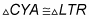Reason for statement 6: AA (If two angles of one triangle are congruent to two angles of another triangle, then the triangles are similar; lines 3 and 5).